# Modbus (RS-485) Using Arduino

We implement RS-485 protocol in communication between two Arduinos using MAX485 module.

IntermediateShowcase (no instructions)1 hour650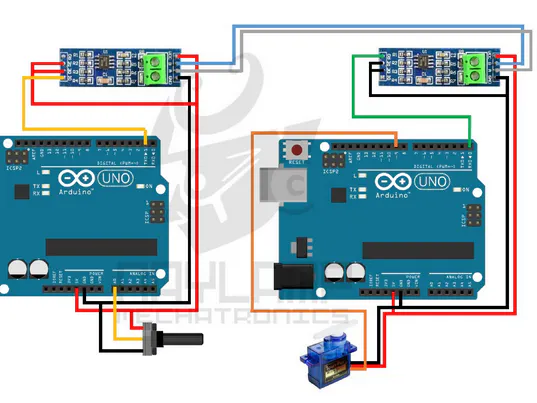## Things used in this project

### Hardware componentsArduino UNO & Genuino UNO
×2
 5V MAX485 TTL to RS485
×1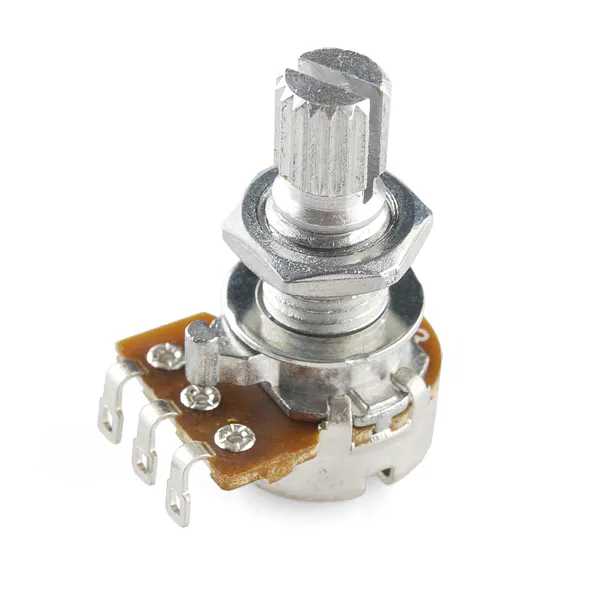Rotary potentiometer (generic)
×2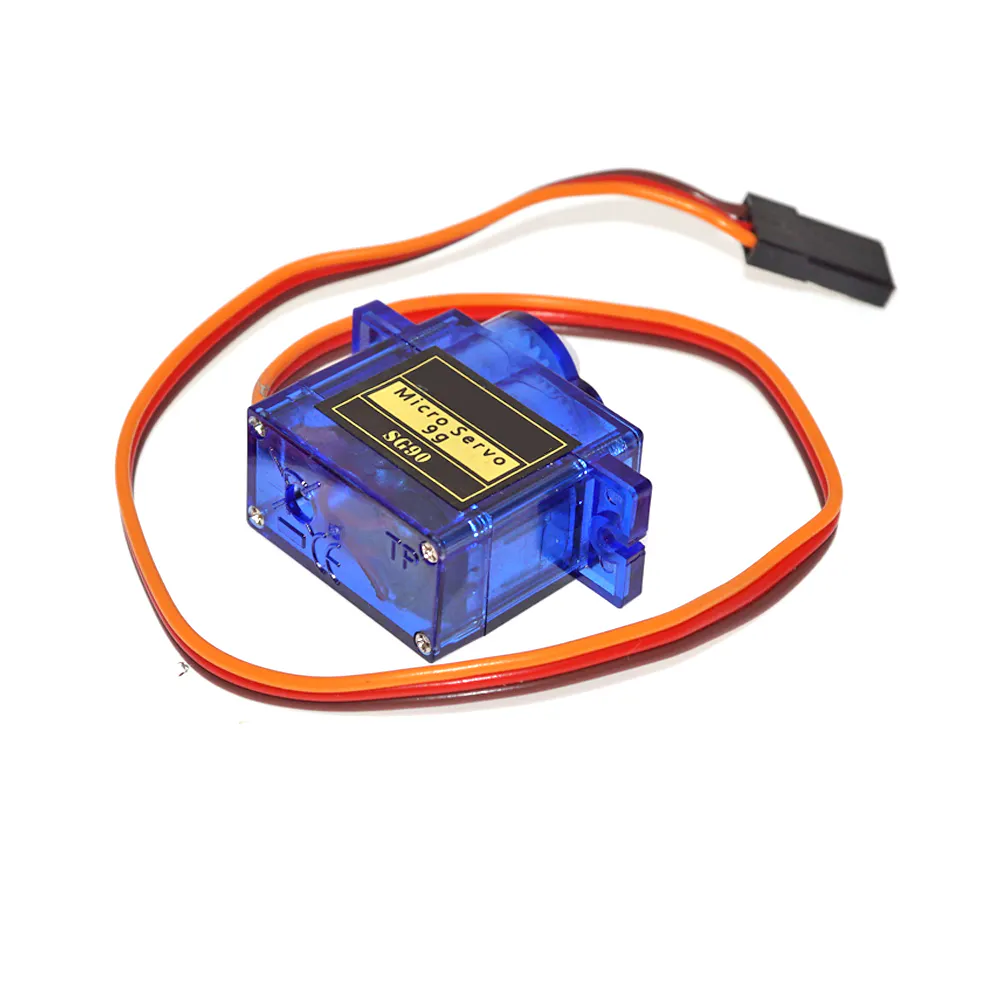SG90 Micro-servo motor
×1×1Jumper wires (generic)
×10

### Software apps and online servicesArduino IDE

## Schematics

### Simplex

For transmitter (Arduino 1)
MAX485 Module -> Arduino
Vcc -> 5V
GND -> GND
RO -> -
RE -> 5V
DE -> 5V
DI -> TX

Potentiometer -> Arduino
Vcc -> 5V
GND -> GND
Output -> A0

MAX485 Module -> Arduino
Vcc -> 5V
GND -> GND
RO -> RX
RE -> GND
DE -> GND
DI -> -

Servo Motor -> Arduino
Vcc (Red) -> 5V
GND (Brown) -> GND
PWM (Orange) -> GPIO 9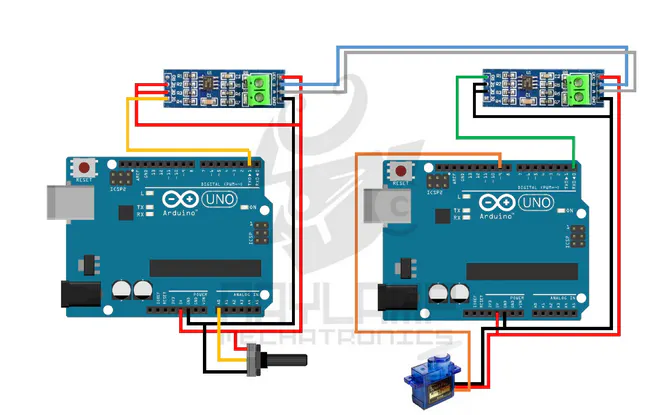### Half Duplex

For both Arduino
MAX485 Module -> Arduino
Vcc -> 5V
GND -> GND
RO -> RX
RE -> GPIO 2
DE -> GPIO 2
DI -> TX

For Arduino 1
Potentiometer -> Arduino
Vcc -> 5V
GND -> GND
Output -> A0

For Arduino 2:
Servo Motor -> Arduino
Vcc (Red) -> 5V
GND (Brown) -> GND
PWM (Orange) -> GPIO 9

Potentiometer -> Arduino (Arduino 2)
Vcc -> 5V
GND -> GND
Output -> A0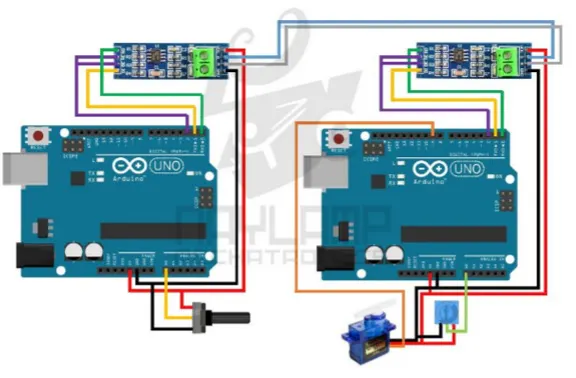## Code

### Peer 1 (Half Duplex)

C/C++
Transmitter data frame
header + identifier + data value + footer

```const int ledPin =  13;  // Built-in LED
const int EnTxPin =  2;  // HIGH:Transmitter, LOW:Receiver
void setup()
{
Serial.begin(9600);
Serial.setTimeout(100);
pinMode(ledPin, OUTPUT);
pinMode(EnTxPin, OUTPUT);
digitalWrite(ledPin, LOW);
digitalWrite(EnTxPin, HIGH);
}

void loop()
{
int rdata = analogRead(0); //data from potentiometer
int angle= map(rdata, 0, 1023, 0, 180);

//transmitter data packet
Serial.print("I"); //initiate data packet
Serial.print("S"); //code for servo
Serial.print(angle); //servo angle data
Serial.print("F"); //finish data packet
delay(50);

Serial.print("I"); //initiate data packet
Serial.print("L"); //code for sensor
Serial.print("F"); //finish data packet
Serial.flush();

if(Serial.find("i"))
{
int data=Serial.parseInt();
{
onLED(data);
}

}
digitalWrite(EnTxPin, HIGH); //RS485 as transmitter

}

void onLED(int data)
{
if(data>500)
digitalWrite(ledPin, HIGH);
else
digitalWrite(ledPin, LOW);
}
```

### Peer 2 (Half Duplex)

C/C++
Transmitter data frame
header + identifier + data value + footer

```#include <Servo.h>
Servo myservo;
const int EnTxPin = 2;
void setup () {
Serial.begin (9600);
myservo.attach (9);
pinMode(EnTxPin, OUTPUT );
digitalWrite (EnTxPin, LOW );
}

void loop (){
if ( Serial.available ()){
if ( Serial.read () == 'I' ){
if (function == 'S' ){
int angle = Serial.parseInt ();
if ( Serial.read () == 'F' ){
if (angle <= 180) {
myservo.write (angle);
}
}
}
else if (function == 'L' ){
if ( Serial.read () == 'F' ){
digitalWrite (EnTxPin, HIGH ); //enable to transmit
Serial.print ( "i" );
Serial.print (val);
Serial.println ( "f" );
Serial.flush ();
digitalWrite (EnTxPin, LOW ); //enable to receive
}
}
}
}
delay (10);
}

#include <Servo.h>
Servo myservo;
const int EnTxPin = 2;
void setup () {
Serial.begin (9600);
myservo.attach (9);
pinMode(EnTxPin, OUTPUT );
digitalWrite (EnTxPin, LOW );
}

void loop (){
if ( Serial.available ()){
if ( Serial.read () == 'I' ){
if (function == 'S' ){
int angle = Serial.parseInt ();
if ( Serial.read () == 'F' ){
if (angle <= 180) {
myservo.write (angle);
}
}
}
else if (function == 'L' ){
if ( Serial.read () == 'F' ){
digitalWrite (EnTxPin, HIGH ); //enable to transmit
Serial.print ( "i" );
Serial.print (val);
Serial.println ( "f" );
Serial.flush ();
digitalWrite (EnTxPin, LOW ); //enable to receive
}
}
}
}
delay (10);
}
```

### Transmitter (Simplex)

C/C++
```void setup()
{
Serial.begin(9600);
}

void loop()
{
byte angle= map(data, 0, 1023, 0, 180);
Serial.write(angle);
delay(50);
}
```

C/C++
```#include <Servo.h>

Servo myservo;

void setup()
{
Serial.begin(9600);
myservo.attach(9);
}

void loop()
{

if (Serial.available()) {